### Ticker

6/recent/ticker-posts

# Regression

The idea behind regression is that when there is significant linear correlation, you can use a line to estimate the value of the dependent variable for certain values of the independent variable.The regression equation should only used
• When there is significant linear correlation. That is, when you reject the null hypothesis that rho=0 in a correlation hypothesis test.
• The value of the independent variable being used in the estimation is close to the original values. That is, you should not use a regression equation obtained using x's between 10 and 20 to estimate y when x is 200.
• The regression equation should not be used with different populations. That is, if x is the height of a male, and y is the weight of a male, then you shouldn't use the regression equation to estimate the weight of a female.
• The regression equation shouldn't be used to forecast values not from that time frame. If data is from the 1960's, it probably isn't valid in the 1990's.
Simple regression is used to examine the relationship between one dependent and one independent variable. After performing an analysis, the regression statistics can be used to predict the dependent variable when the independent variable is known. Regression goes beyond correlation by adding prediction capabilities.
People use regression on an intuitive level every day. In business, a well-dressed man is thought to be financially successful. A mother knows that more sugar in her children's diet results in higher energy levels. The ease of waking up in the morning often depends on how late you went to bed the night before. Quantitative regression adds precision by developing a mathematical formula that can be used for predictive purposes.
For example, a medical researcher might want to use body weight (independent variable) to predict the most appropriate dose for a new drug (dependent variable). The purpose of running the regression is to find a formula that fits the relationship between the two variables. Then you can use that formula to predict values for the dependent variable when only the independent variable is known. A doctor could prescribe the proper dose based on a person's body weight.
The regression line (known as the least squares line) is a plot of the expected value of the dependent variable for all values of the independent variable. Technically, it is the line that "minimizes the squared residuals". The regression line is the one that best fits the data on a scatterplot.
Using the regression equation, the dependent variable may be predicted from the independent variable. The slope of the regression line (b) is defined as the rise divided by the run. The y intercept (a) is the point on the y axis where the regression line would intercept the y axis. The slope and y intercept are incorporated into the regression equation. The intercept is usually called the constant, and the slope is referred to as the coefficient. Since the regression model is usually not a perfect predictor, there is also an error term in the equation.
In the regression equation, y is always the dependent variable and x is always the independent variable. Here are three equivalent ways to mathematically describe a linear regression model.
y = intercept + (slopex) + error
y = constant + (coefficientx) + error
y = a + bx + e
The significance of the slope of the regression line is determined from the t-statistic. It is the probability that the observed correlation coefficient occurred by chance if the true correlation is zero. Some researchers prefer to report the F-ratio instead of the t-statistic. The F-ratio is equal to the t-statistic squared.
The t-statistic for the significance of the slope is essentially a test to determine if the regression model (equation) is usable. If the slope is significantly different than zero, then we can use the regression model to predict the dependent variable for any value of the independent variable.
On the other hand, take an example where the slope is zero. It has no prediction ability because for every value of the independent variable, the prediction for the dependent variable would be the same. Knowing the value of the independent variable would not improve our ability to predict the dependent variable. Thus, if the slope is not significantly different than zero, don't use the model to make predictions.
The coefficient of determination (r-squared) is the square of the correlation coefficient. Its value may vary from zero to one. It has the advantage over the correlation coefficient in that it may be interpreted directly as the proportion of variance in the dependent variable that can be accounted for by the regression equation. For example, an r-squared value of .49 means that 49% of the variance in the dependent variable can be explained by the regression equation. The other 51% is unexplained.
The standard error of the estimate for regression measures the amount of variability in the points around the regression line. It is the standard deviation of the data points as they are distributed around the regression line. The standard error of the estimate can be used to develop confidence intervals around a prediction.
Example
A company wants to know if there is a significant relationship between its advertising expenditures and its sales volume. The independent variable is advertising budget and the dependent variable is sales volume. A lag time of one month will be used because sales are expected to lag behind actual advertising expenditures. Data was collected for a six month period. All figures are in thousands of dollars. Is there a significant relationship between advertising budget and sales volume?
 Indep. Var. Depen. Var 4.2 27.1 6.1 30.4 3.9 25.0 5.7 29.7 7.3 40.1 5.9 28.8
--------------------------------------------------
Model: y = 9.873 + (3.682x) + error
Standard error of the estimate = 2.637
t-test for the significance of the slope = 3.961
Degrees of freedom = 4
Two-tailed probability = .0149
r-squared = .807
You might make a statement in a report like this: A simple linear regression was performed on six months of data to determine if there was a significant relationship between advertising expenditures and sales volume. The t-statistic for the slope was significant at the .05 critical alpha level, t(4)=3.96, p=.015. Thus, we reject the null hypothesis and conclude that there was a positive significant relationship between advertising expenditures and sales volume. Furthermore, 80.7% of the variability in sales volume could be explained by advertising expenditures.

Assuming that you've decided that you can have a regression equation because there is significant linear correlation between the two variables, the equation becomes:y' = ax + b or y' = a + bx (some books use y-hat instead of y-prime). The Bluman text uses the second formula, however, more people are familiar with the notion of y = mx + b, so I will use the first.
a is the slope of the regression line:b is the y-intercept of the regression line:The regression line is sometimes called the "line of best fit" or the "best fit line".
Since it "best fits" the data, it makes sense that the line passes through the means.
The regression equation is the line with slope a passing through the point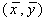Another way to write the equation would be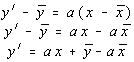apply just a little algebra, and we have the formulas for a and b that we would use (if we were stranded on a desert island without the TI-82) ...
It also turns out that the slope of the regression line can be written as. Since the standard deviations can't be negative, the sign of the slope is determined by the sign of the correlation coefficient. This agrees with the statement made earlier that the slope of the regression line will have the same slope as the correlation coefficient.

## Scatter Plots

1. Enter the x values into L1 and the y variables into L2.
2. Go to Stat Plot (2nd y=)
3. Turn Plot 1 on
4. Choose the type to be scatter plot (1st type)
5. Set Xlist to L1
6. Set Ylist to L2
7. Set the Mark to any of the three choices
8. Zoom to the Stat setting (#9)
Note, the Ylist and Mark won't show up until you select a scatter plot

## Regression Lines

1. Setup the scatter plot as instructed above
2. Go into the Stats, Calc, Setup screen
3. Setup the 2-Var Stats so that: Xlist = L1, Ylist = L2, Freq = 1
4. Calculate the Linear Regression (ax+b) (#5)
5. Go into the Plot screen.
6. Position the cursor on the Y1 plot and hit CLEAR to erase it.
7. While still in the Y1 data entry field, go to the VARS, STATS, EQ screen and choose option 7 which is the regression equation
8. Hit GRAPH

## Regression Lines, part 2

The above technique works, but it requires that you change the equation being graphed every time you change problems. It is possible to stick the regression equation "ax+b" into the Y1 plot and then it will automatically graph the correct regression equation each time.

### Do this once

1. Setup the scatter plot as instructed above
2. Go into the Plot screen.
3. Position the cursor on the Y1 plot and hit CLEAR to erase it.
4. Enter a*x+b into the function. The a and b can be found under the VARS, STATS, EQ screen

### Do this for each graph

1. Go into the Stats, Calc, Setup screen
2. Setup the 2-Var Stats so that: Xlist = L1, Ylist = L2, Freq = 1
3. Calculate the Linear Regression (ax+b) (#5)
4. Hit the GRAPH key
It is important that you calculate the linear regression variables before trying to graph the regression line. If you change the data in the lists or have not calculated the linear regression equations, then you will get an " ERR: Undefined" when you try to graph the data.
Be sure to turn off the stats plots and/or the Y1 plot when you need to graph other data

.Standard Error of EstimateLet us consider yest as the estimated value of y for a given value of x. This estimated value can be obtained from the regression curve of y on x (seeLinear regression ). From this, the measure of the scatter about the regression curve is supplied by the quantity:The above equation is called the Standard Error of Estimate of y on x. It is important to note that this Standard Error of Estimate has properties analogous to those of standard deviation.Example:

The regression line of y on x is given by the equation:
y = 35.82 + 0.476 x
The following table depicts the actual values of y and the estimated values of y (denoted by yest).
x65636764
y68666865
yest66.7665.8167.7166.28
y - yest1.240.190.29-1.28

Applying the formula for the Standard Error Estimate for this problem we have the solution as follows.Correlation Coefficient
How well does your regression equation truly represent

One of the ways to determine the answer to this question is to
exam the  correlation coefficient and the coefficient of determination.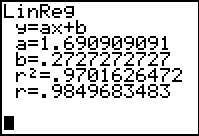The correlation coefficient, r, and the coefficient of determination, r 2 ,will appear on the screen that shows the regression equation information (be sure the Diagnostics are turned on ---2nd Catalog (above 0), arrow down toDiagnosticOn, press ENTER twice.)

 In addition to appearing with the regression information, the values r and r 2 can be found under VARS, #5 Statistics → EQ #7 r and #8 r 2 .

 Correlation Coefficient, r :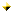The quantity r, called the linear correlation coefficient, measures the strength and
the direction of a linear relationship between two variables.
The linear correlation
coefficient is sometimes referred to as the Pearson product moment correlation coefficient in
honor of its developer Karl Pearson.The mathematical formula for computing r is: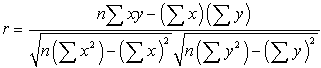where n is the number of pairs of data.
(Aren't you glad you have a graphing calculator that computes this formula?)The value of r is such that -1 < r < +1.  The + and – signs are used for positive
linear correlations and negative linear correlations, respectively.Positive correlation:    If x and y have a strong positive linear correlation, r is close
to +1.  An r value of exactly +1 indicates a perfect positive fit.
Positive values
indicate a relationship between x and y variables such that as values for x increases,
values for  also increase.Negative correlation:   If x and y have a strong negative linear correlation, r is close
to -1.  An r value of exactly -1 indicates a perfect negative fit.
Negative values
indicate a relationship between x and such that as values for x increase, values
for y decrease.No correlation:  If there is no linear correlation or a weak linear correlation, r is
close to 0.
A value near zero means that there is a random, nonlinear relationship
between the two variablesNote that r is a dimensionless quantity; that is, it does not depend on the units
employed.perfect correlation of ± 1 occurs only when the data points all lie exactly on a
straight line.  If r = +1, the slope of this line is positive.  If r = -1, the slope of this
line is negative.A correlation greater than 0.8 is generally described as strong, whereas a correlation
less than 0.5 is generally described as weak.
These values can vary based upon the
"type" of data being examined.  A study utilizing scientific data may require a stronger
correlation than a study using social science data.

 Coefficient of Determination, r 2  or  R2 :The coefficient of determination, 2, is useful because it gives the proportion of
the variance (fluctuation) of one variable that is predictable from the other variable
.

It is a measure that allows us to determine how certain one can be in making
predictions from a certain model/graph.The coefficient of determination is the ratio of the explained variation to the total
variation.The coefficient of determination is such that 0 <  r 2 < 1,  and denotes the strength
of the linear association between x and yThe coefficient of determination represents the percent of the data that is the closest
to the line of best fit.  For example, if r = 0.922, then 2 = 0.850, which means that
85% of the total variation in y can be explained by the linear relationship between x

and y (as described by the regression equation).  The other 15% of the total variation
in y remains unexplained.The coefficient of determination is a measure of how well the regression line
represents the data.  If the regression line passes exactly through every point on the
scatter plot, it would be able to explain all of the variation. The further the line is
away from the points, the less it is able to explain.